# Calculator

sprinkles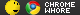2009 Sep 6 • 2547
10 ₧
So as you can guess I am making a calculator. Its a kinda advance calculator it can do quadratics and polynomials and such.
right now I am working with the quadratic equation.
i have it working fine for positive numbers so i figured i could
jus copy and paste the code but invert it [make + into - and such]
but apparently i can't currently the code is
Visual Basic code
Public Function calcequation() Dim first As String = "" Dim sec As Double Dim firstop As String = "" Dim secop As String = "" Dim third As String = "" Dim fourth As Double If a = 1 Then first = "x" third = "x" ElseIf a <> 1 Then a = a / 2 End If If a = 1 Then first = "x" third = "x" ElseIf a <> 1 Then first = a.ToString() + "x" third = a.ToString() + "x" End If If b > 0 And c > 0 Then firstop = "+" secop = "+" If c <= 100 And c > 0 Then Do Until sec * fourth = c And sec + fourth = b If fourth / 10 = 1 Or fourth / 10 = 2 Or fourth / 10 = 3 Or fourth / 10 = 4 Or fourth / 10 = 5 Or fourth / 10 = 6 Or fourth / 10 = 7 Or fourth / 10 = 8 Or fourth / 10 = 9 Or fourth / 10 = 10 Then sec = sec + 1 fourth = 0 End If fourth = fourth + 1 Loop End If If c <= 200 And c > 100 Then Do Until sec * fourth = c And sec + fourth = b If fourth / 10 = 11 Or fourth / 10 = 12 Or fourth / 10 = 13 Or fourth / 10 = 14 Or fourth / 10 = 15 Or fourth / 10 = 16 Or fourth / 10 = 17 Or fourth / 10 = 18 Or fourth / 10 = 19 Or fourth / 10 = 20 Then sec = sec + 1 fourth = 0 End If fourth = fourth + 1 Loop End If End If If b > 0 And c < 0 Then End If If b < 0 And c > 0 Then If b >= -100 And b < 0 Then Do Until sec * fourth = c And sec + fourth = b If fourth / 10 = -1 Or fourth / 10 = -2 Or fourth / 10 = -3 Or fourth / 10 = -4 Or fourth / 10 = -5 Or fourth / 10 = -6 Or fourth / 10 = -7 Or fourth / 10 = -8 Or fourth / 10 = -9 Or fourth / 10 = -10 Then sec = sec - 1 fourth = 0 End If fourth = fourth - 1 Loop End If If b >= -200 And b < -100 Then Do Until sec * fourth = c And sec + fourth = b If fourth / 10 = -11 Or fourth / 10 = -12 Or fourth / 10 = -13 Or fourth / 10 = -14 Or fourth / 10 = -15 Or fourth / 10 = -16 Or fourth / 10 = -17 Or fourth / 10 = -18 Or fourth / 10 = -19 Or fourth / 10 = -20 Then sec = sec - 1 fourth = 0 End If fourth = fourth - 1 Loop End If End If If sec < 0 Then secabsolute = Math.Abs(sec) If fourth < 0 Then fourthabsolute = Math.Abs(fourth) If sec > 0 Then Dim num As Integer num = sec * 2 secabsolute = sec - num End If If fourth > 0 Then Dim num As Integer num = fourth * 2 fourthabsolute = fourth - num End If x = secabsolute.ToString() + " or x= " + fourthabsolute.ToString() '"(" + first + firstop + sec.ToString() + ")(" + third + secop + fourth.ToString() + ")" Return x End Function

Please don't tell me [right now at least] that there is an easier/better way to do it. I want to know for now why when i put a negative value in b or c it doesnt work [it freezes and maxes out my cpu] the values i tried are A=1 B=24 C=144 and A=1 B=13 C=42 [both are quadratic because when i put them in as positives they give my 12,12 and 6,7)but making either B or C negative [not both neg at the same time because i don't believe you can do that can you?] I will step into the code to see what its freezing on [obviously its going to be a do...loop but which one]≡ 2009 Dec 20 at 12:16 PST — Ed. 2009 Dec 20 at 12:27 PSTthe_cloud_system
polly pushy pants2008 Aug 1 • 3076
-6 ₧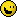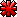I drink to forget but I always remember.
 ≡ 2009 Dec 20 at 12:27 PSTDown Rodeo
Cap'n Moth of the Firehouse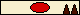2007 Oct 19 • 5486
57,583 ₧
Righto, quadratics. I assume you're using the form a*x^2 + b*x + c = 0, if you're not, well, you should be. Every quadratic equation will have two solutions that satisfy the above equation. The problem you're running into, I think, is where b^2 - 4*a*c is less than zero. How much do you know about complex numbers? Essentially, the values you want are given by

(-b
 ≡ 2009 Dec 20 at 13:47 PSTsprinkles2009 Sep 6 • 2547
10 ₧
Nooooooooooo. I was factoring the quadratic equation not using the formula. You know that whole thing about x^2+13x+42=(x+6)(x+7)

The quadratic formula works fine [well actually i dont think i tried negs in it]

ironically i thought the formula would be hard and the equation easy
WRONG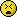ok jus checked the formula with a=1 b=-13 c=42 got 0.5 and -0.5
which means that there is something wrong mathmatically witch
shouldn't be to hard to fix≡ 2009 Dec 20 at 13:51 PST — Ed. 2009 Dec 20 at 13:55 PSTDown Rodeo
Cap'n Moth of the Firehouse2007 Oct 19 • 5486
57,583 ₧
They're essentially the same thing... try the equation for finding the roots on the example you gave, you'll end up with -6 and -7, I bet you.
 ≡ 2009 Dec 20 at 13:56 PSTsprinkles2009 Sep 6 • 2547
10 ₧
Nope with the equation i get a maxed out cpu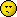let me clean up my code so I can actually understand it.
Then I will step into it again and see whats wrong [maybe]≡ 2009 Dec 20 at 13:59 PSTDown Rodeo
Cap'n Moth of the Firehouse2007 Oct 19 • 5486
57,583 ₧
This code works:
java code
cat SciProg/Checkpoint4_1.java // Calculates square roots import java.io.Console; // For console IO public class Checkpoint4_1 { /*private static double read_double() { double ret; boolean done; do { done = true; try { ret = new Double(c.readLine()); } catch ( NumberFormatException e ) { done = false; } } while ( !done ) ; return ret; }*/ public static void main(String[] args) { double a,b,c,x_one,x_two,disc; // Declaring variables for later use - the coefficients, x co-ordinates and discriminant. String temp; // For input from console. Console myConsole = System.console(); // Initialise console as currently used console. // Explanatory text follows. myConsole.printf("This program will take three arguments from the command line: the coefficients\na,b and c in the equation ax^2 + bx + c = 0.\ It will then print out the roots of the quadratic (or linear) equation.\n"); temp = myConsole.readLine(); // These six lines take a string from the terminal then attempt to read a Double from it. a = new Double(temp); // Will die if not able to read one properly but input sanitisation hardly seems necessary. temp = myConsole.readLine(); b = new Double(temp); temp = myConsole.readLine(); c = new Double(temp); if(a!=0) // Checks if we have a quadratic. { disc = b*b - 4*a*c; // Sets discriminant. if(disc > 0) // Two roots. { x_one = (-1*b - Math.sqrt(disc))/2*a; x_two = (-1*b + Math.sqrt(disc))/2*a; myConsole.printf("The quadratic has two roots at %.2f and %.2f.\n",x_one,x_two); } else if(disc == 0) // Single root. { x_one = (-1*b)/(2*a); myConsole.printf("The quadratic has a double root at %.2f.\n",x_one); } else // Complex roots. { x_one = (-1*b)/(2*a); x_two = Math.sqrt(-1*disc)/(2*a); myConsole.printf("The quadratic has two complex roots at %.2f - %.2fi and %.2f + %.2fi.\n",x_one,x_two,x_one,x_two); } } else if(b!=0) // If it's not a quadratic, is it linear? { x_one = -1*c/b; myConsole.printf("This linear equation has a root at %.2f.\n",x_one); } else // If not that then it must be constant. { myConsole.printf("This is a constant equation and has no roots.\n"); } System.exit(0); // Time to go. } }

Java syntax is easy enough to understand, and I've commented it quite well.
 ≡ 2009 Dec 20 at 14:08 PST — Ed. 2009 Dec 20 at 14:09 PSTsprinkles2009 Sep 6 • 2547
10 ₧
I got the formula working. The problem is that I was dividing
the end result instead of outputting it as number/number
[a fraction]. Now the problem is that I have to figure out
how I can reduce the fraction. For a=1 b=13 c=42 I get 12/2
and 14/2. To reduce the fractions you have to have a number
that can divide into both of the numbers right? Fuck I hate math.

Ughhh back to gcf.≡ 2009 Dec 20 at 14:56 PST — Ed. 2009 Dec 20 at 14:58 PSTDown Rodeo
Cap'n Moth of the Firehouse2007 Oct 19 • 5486
57,583 ₧
Let's assume you have a function that returns all the factors of a number - this will be useful for your calculator anyway. Have it return them as a list (of integers). Have another that returns the list sans 1 and the number passed in. You could call them "factors_i" and "factors_e" for inclusive and exclusive, I suppose.

code
for(int j = 0; j < length; ++j) { if(denom % factors[j] == 0) denom /= factors[j]; }

This is probably not Basic-like but again it ought to be an easy change. The percent sign is modulus, the /= means divide left by right and assign it to left, factors[j] is assumed to have been assigned by int []factors = factors_e(numerator); then length would be length(factors). Yes?

PS Soopyjams, I founds an error in your softwares! Or, something that doesn't necessarily work as expected.
 ≡ 2009 Dec 20 at 15:22 PST — Ed. 2009 Dec 20 at 15:51 PSTsprinkles2009 Sep 6 • 2547
10 ₧
But GCF only cares about the prime number factors.
I got it to reduce, 24 is now 12, but 12 has to be 6 and then 6 has to be 3 which means more code unless I can figure out how to put this in an for statement....≡ 2009 Dec 20 at 16:10 PST — Ed. 2009 Dec 20 at 16:14 PSTDown Rodeo
Cap'n Moth of the Firehouse2007 Oct 19 • 5486
57,583 ₧
Ok, I should have said prime number factors, you're right. So make a function that returns the prime number factors.
 ≡ 2009 Dec 20 at 16:14 PSTDown Rodeo
Cap'n Moth of the Firehouse2007 Oct 19 • 5486
57,583 ₧
What's GCF? Also, the code I posted will loop, and if they share any common factors, it will divide them out.
 ≡ 2009 Dec 20 at 17:37 PSTsprinkles2009 Sep 6 • 2547
10 ₧
Greatest Common Factor≡ 2009 Dec 21 at 10:27 PSTMate de Vita
Kelli2008 Oct 4 • 2453
159 ₧
Down Rodeo said:
PS Soopyjams, I founds an error in your softwares! Or, something that doesn't necessarily work as expected.

And what be that error, matey?
...and that's the bottom line because Mate de Vita said so.
 ≡ 2009 Dec 21 at 11:03 PSTDown Rodeo
Cap'n Moth of the Firehouse2007 Oct 19 • 5486
57,583 ₧
Try writing the for loop without any spaces.
 ≡ 2009 Dec 21 at 13:27 PSTSuperJer
Websiteman2005 Mar 20 • 6349
Down Rodeo said:
Try writing the for loop without any spaces.

Like this?
code
for(int j=0;j<

Good catch. Thanks. I fixed it now.
 ≡ 2009 Dec 21 at 15:01 PST — Ed. 2009 Dec 21 at 15:12 PSTDown Rodeo
Cap'n Moth of the Firehouse2007 Oct 19 • 5486
57,583 ₧
Wow, you're a hero. Any thoughts on the bots issue? Since that thread has been largely hijacked.
 ≡ 2009 Dec 21 at 16:19 PSTsprinkles2009 Sep 6 • 2547
10 ₧
I am going to try the For each statement with an array of prime
numbers [ending at 13]. The problem is I can't jus' do it once.
For example if I have 24/2 I have to reduce 24 to 12 to 6 to 3.
I need a general statement that will let me continue reducing
until it is fully reduced without knowing how many times I have
to go through that process again [and then I have to do it for
the second value of x and then for the denominator of each]

Ok, so I jus' whipped something up and its

[your code tag is broken?]
For Each number As Integer in Prime
remainder=IEEERemainer(num1,number) 'divide num1 by number [a value in the array Prime] and set remainder to the left over value
If remainder=0 Then 'if there is no remainder
firstDivisor=number 'save that number to firstDivisor do divide by later
Exit For 'don't complete the loop [because it may be divisible by another number which wouldnt work
End If
Next
Dim divisor2 As Integer
[/code is it still broken?]

Now it's important to note that that finds the greatest
common factor, but it does not yet divide by the gcf.
Thus, it saves the gcf in firstDivisor.

Oh the code tag is broken because I put [] in it≡ 2009 Dec 21 at 18:06 PST — Ed. 2009 Dec 21 at 18:27 PSTDown Rodeo
Cap'n Moth of the Firehouse2007 Oct 19 • 5486
57,583 ₧
Down Rodeo said:
code
for(int j = 0; j < length; ++j) { if(denom % factors[j] == 0) denom /= factors[j]; }

Stupid stupid stupid! Needs to be more like:
code
for(int j = 0; j < length; ++j) { while(denom % factors[j] == 0) { denom /= factors[j]; } }
 ≡ 2009 Dec 21 at 18:29 PSTsprinkles2009 Sep 6 • 2547
10 ₧
Oh by the way what java compiler do you use/recommend?

I am using Bluej right now because that is what we used in java
class [at least for the 1/5 of the class I stayed for they could
have switched to something else as soon as I left].

Well I don't know if that For Each statement works yet, but it
replaces 32 lines of code!

Ok I found a way to do it all in a Do While statement containing
2 For Each statements the problem is that I need to actively
modify the array inside the For Each statement this is a
problem, but microsoft said if you use the IEnumerable thingy
you can do it without getting a run time error. However, they
say that you really need to know IEnumerable to use it
correctly, and I don't understand it [because they don't explain
it] at all. So if someone would be nice enough to simplify
me to understand......Thank you.

I dont know what this is......
code
Imports Microsoft.Win32 Imports System.IO Imports System.Math Public Class Form1 Dim num1 As Double = 24 Dim Prime() As Integer = {2, 3, 5, 7, 11, 13} Dim reducenum1Array() As Integer = {num1} Dim divisorArray() As Integer = {} Dim Toreducenum1Array As Integer Dim TodivisorArray As Integer Dim remainder As Double Dim complete As Boolean = False Dim newsize As Integer = 2 Dim newsize1 As Integer = 1 Dim out As Integer Public Sub reducenum1() for1: For Each temp As Integer In reducenum1Array Toreducenum1Array = temp setvalue_reducenum1Array() for2: For Each number As Integer In Prime remainder = IEEERemainder(temp, number) If remainder = 0 Then TodivisorArray = number setvalue_divisorArray() If number = 13 Then complete = True Exit Sub End If Next number GoTo for2 Next temp GoTo for1 End Sub Public Sub setvalue_reducenum1Array() Array.Resize(reducenum1Array, newsize) reducenum1Array.SetValue(Toreducenum1Array, newsize1) End Sub Public Sub setvalue_divisorArray() End Sub Private Sub Button2_Click(ByVal sender As System.Object, ByVal e As System.EventArgs) Handles Button2.Click Application.Exit() End Sub Private Sub Button1_Click(ByVal sender As System.Object, ByVal e As System.EventArgs) Handles Button1.Click reducenum1() ' setvalue_reducenum1Array() setvalue_divisorArray() For i As Integer = 0 To newsize Dim value As Integer value += 1 Label2.Text = Label2.Text + reducenum1Array.GetValue(value).ToString() Next End Sub End Class

Ok, so the problem is the array isn't getting bigger.

No disregard that code I get an array of 24,12,0.
its not perfect but its not 242424 or anything weird like that.

FUCK ARRAYS
code
Public Sub reducenum1() for1: For Each number As Integer In Prime remainder = IEEERemainder(temp, number) If remainder = 0 Then value = temp / number Label2.Text = Label2.Text + value.ToString() temp = value GoTo for1 End If If number = 13 Then complete = True Exit Sub End If Next number End Sub

That does it [and I know that 'real' programmers shouldn't
use the GoTo command but what the hell.≡ 2009 Dec 21 at 18:32 PST — Ed. 2009 Dec 21 at 23:01 PSTDown Rodeo
Cap'n Moth of the Firehouse2007 Oct 19 • 5486
57,583 ₧
I'm not sure as I don't understand Basic all that well. It's been too long and I'm not used to writing in that style (I try to cut things down). I know I can barely criticise your lack of comments seeing as my little loop there has none either but it makes it very difficult to know what each line is supposed to do. To make it easier the code I posted loops over each factor in the list (should be the prime factors) then while the number to be reduced divided by the factor has no remainder, divides by it. Of course you could make them both while loops but I think this keeps it clear. Also I forgot to put a tally of what the numerator should be, but it'd be the same line just below the one that says denom.
 ≡ 2009 Dec 22 at 03:37 PSTsprinkles2009 Sep 6 • 2547
10 ₧
Well the code isn't finished yet, because it does not account for
the denominator. So as soon as I get it to account for the
denominator and it works in context I will comment the hell out
of it and post it.≡ 2009 Dec 22 at 06:22 PSTDown Rodeo
Cap'n Moth of the Firehouse2007 Oct 19 • 5486
57,583 ₧
Fair enough. As for the compiler... I use whatever the default is, I think. Probably the sun one.
 ≡ 2009 Dec 22 at 06:29 PSTsprinkles2009 Sep 6 • 2547
10 ₧
here it is DR!

code
Public Sub reducenum1() 'enters the sub routine reduce num1 temp = num1 'sets the value of temp [currently Nothing] to the value of num1 [currently x1] for1: For Each number As Integer In Prime 'for numbers 2,3,5,7,11,13 do whats next remainder = IEEERemainder(temp, number) 'set remainder to the value of the left over [ie remainder] of temp divided by number If remainder = 0 Then 'if there is no remiander [it divides evenly into temp thus giving you the gcf of temp] remainder = IEEERemainder(temp2, number) 'set remainder to the value of temp2 [the denominator of x1 and x2] divded by number If remainder = 0 Then 'if there is no left over value2 = temp2 / number 'divid temp2 [denominator of x1 and x2] set value to value2 value = temp / number 'do the same as above except for the nominator of x1 valuestore = valuestore + "-" + value.ToString() 'set the new value of the nominator of x1 to a string seperating numbers by a - temp = value 'sets temp to the value of temp/number [ie emulating an array in the For Each loop temp2 = value2 christ = number GoTo for1 'repeat the process over and over [it does this because there is still a possibility of reducing the nominator AND denominator of x1] End If End If If number = 13 Then 'if number=13 [ie you cannot divide by the first 6 prime numbers exit loop complete = True Exit Sub End If Next number End Sub Public Function findnumber1() Dim length As Integer value = temp / christ valuestore = valuestore + "-" + value.ToString() length = valuestore.LastIndexOf("-") bob = valuestore.Remove(length) length = bob.LastIndexOf("-") length += 1 jesus = bob.Remove(0, length) Return jesus End Function '''<summary> ''' the above function does some fancy stuff ''' first of all it fixes the error that the For Each loop does not account for that ''' that is the fact that the For Each loop divides by the number of indexes of denominator ''' so 8=2x2x2 [3 indexs] it is important to note that 8 is the sample denominator ''' (64/2) does that 3 times which results in 16 ''' however 16/1 is NOT a reduction of 64/8 ''' 8/1 is the fully reduced 64/8 ''' we get this by dividing outside the for statement ''' one last time ''' then it finds the last index the - ''' and deletes anything after the last - ''' then it finds the last index of - again ''' the difference is that at the begining there is a - after the actual number you want ''' the second index of - gets the index of the - before the number you want ''' and the first last index of - deletes from the - to the end ''' the second last index of deletes from the begining to the- ''' which is a problem because -8 is not the answer we want ''' so we add 1 to the last index of - so we delete upto the number ''' you want not the - ''' and all of this is repeated for num2 '''</summary>≡ 2009 Dec 22 at 07:45 PST — Ed. 2009 Dec 22 at 07:49 PSTDown Rodeo
Cap'n Moth of the Firehouse2007 Oct 19 • 5486
57,583 ₧
Ah, um, yes? :p

I'm sure it makes sense. Your code looks extensible as well, for instance, the prime numbers could
be replaced by a more complete list. If you restructure a bit you can remove the goto.
 ≡ 2009 Dec 22 at 09:27 PST — Ed. 2009 Dec 22 at 09:27 PSTPage  2